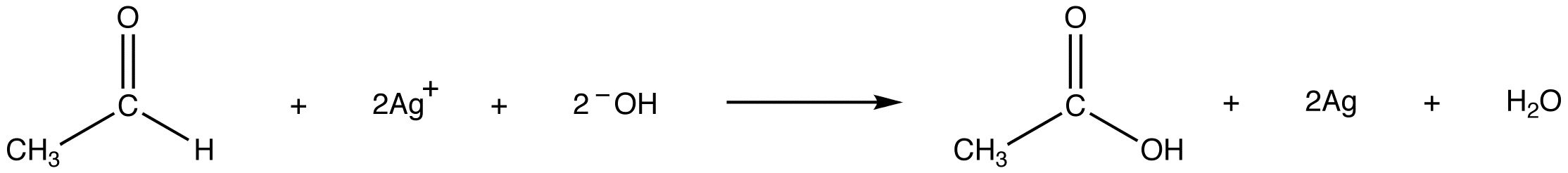# Tollens’ Test

$$\newcommand{\vecs}{\overset { \rightharpoonup} {\mathbf{#1}} }$$ $$\newcommand{\vecd}{\overset{-\!-\!\rightharpoonup}{\vphantom{a}\smash {#1}}}$$$$\newcommand{\id}{\mathrm{id}}$$ $$\newcommand{\Span}{\mathrm{span}}$$ $$\newcommand{\kernel}{\mathrm{null}\,}$$ $$\newcommand{\range}{\mathrm{range}\,}$$ $$\newcommand{\RealPart}{\mathrm{Re}}$$ $$\newcommand{\ImaginaryPart}{\mathrm{Im}}$$ $$\newcommand{\Argument}{\mathrm{Arg}}$$ $$\newcommand{\norm}{\| #1 \|}$$ $$\newcommand{\inner}{\langle #1, #2 \rangle}$$ $$\newcommand{\Span}{\mathrm{span}}$$ $$\newcommand{\id}{\mathrm{id}}$$ $$\newcommand{\Span}{\mathrm{span}}$$ $$\newcommand{\kernel}{\mathrm{null}\,}$$ $$\newcommand{\range}{\mathrm{range}\,}$$ $$\newcommand{\RealPart}{\mathrm{Re}}$$ $$\newcommand{\ImaginaryPart}{\mathrm{Im}}$$ $$\newcommand{\Argument}{\mathrm{Arg}}$$ $$\newcommand{\norm}{\| #1 \|}$$ $$\newcommand{\inner}{\langle #1, #2 \rangle}$$ $$\newcommand{\Span}{\mathrm{span}}$$$$\newcommand{\AA}{\unicode[.8,0]{x212B}}$$

Tollens’ test, also known as silver-mirror test, is a qualitative laboratory test used to distinguish between an aldehyde and a ketone. It exploits the fact that aldehydes are readily oxidized (see oxidation), whereas ketones are not. Tollens’ test uses a reagent known as Tollens’ reagent, which is a colorless, basic, aqueous solution containing silver ions coordinated to ammonia $$[Ag(NH_3)^{2+}]$$. It is prepared using a two-step procedure.

Step 1: Aqueous silver nitrate is mixed with aqueous sodium hydroxide.

\begin{align*} \ce{AgNO_3 + NaOH} &\rightarrow \ce{AgOH + NaHO_3} \\[4pt] \ce{2AgOH} &\rightarrow \ce{Ag_2O + H_2O} \end{align*}

Step 2: Aqueous ammonia is added drop-wise until the precipitated silver oxide completely dissolves.

$\ce{Ag_2O + 4NH_3 + H_2O \rightarrow 2Ag(NH_3)_2^+ + 2OH^{-}} \nonumber$

Tollens’ reagent oxidizes an aldehyde into the corresponding carboxylic acid.The reaction is accompanied by the reduction of silver ions in Tollens’ reagent into metallic silver, which, if the test is carried out in a clean glass test tube, forms a mirror on the test tube. eg:Figure $$\PageIndex{1}$$: Tollens' test for aldehyde: left side positive (silver mirror), right side negative

Ketones are not oxidized by Tollens’ reagent, so the treatment of a ketone with Tollens’ reagent in a glass test tube does not result in a silver mirror (Figure $$\PageIndex{1}$$; right).

## Contributors

Tollens’ Test is shared under a CC BY-NC-SA 4.0 license and was authored, remixed, and/or curated by LibreTexts.